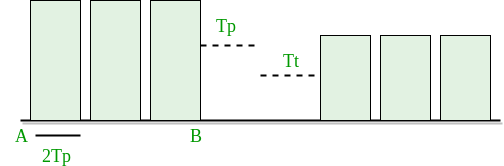# Efficiency of CSMA/CD

• Difficulty Level : Medium
• Last Updated : 06 Apr, 2022

Prerequisite – Introduction to Ethernet, Basics of CSMA/ CD Carrier sense multiple access with collision detection (CSMA/CD) – The CSMA method does not tell us what to do in case there is a collision. Carrier sense multiple access with collision detection (CSMA/CD) adds to the CSMA algorithm to deal with the collision. In CSMA/CD, the size of a frame must be large enough so that collision can be detected by the sender while sending the frame. So, the frame transmission delay must be at least two times the maximum propagation delay. Assume some station transmitted data packet and successfully get to the destination but it is just the Best Case, so we have to take the Worst Case scenario in which there will be contention slots. Contention slots are those slots that are not able to transmit their journey due to the collision. Suppose A station transmitted data but collide and the worst-case time wasted is 2Tp and then some station B found out a way to transmit the data so it took (As shown in Figure)

`Tp ( propagation delay) + Tt(transmission time)`

Now we don’t know how many contention slots, so we consider the worst-case to be of n contention slots.

```Efficiency = Tt / ( C*2*Tp + Tt + Tp)
Tt ? transmission time
Tp ? propagation time
C  ? number of collision```In CSMA/CD, for success, only 1 station should transmit while others shouldn’t. Let p be the probability to transmit data successfully.

`P(success) = nC1 * p * (1-p)n-1 (by using Binomial distribution)`

For max P(success), differentiate with respect to p and equate to zero (to get maxima and minima).

`We get P(max) = 1/e `

Number of times we need to try before getting 1st success

`1/P(MAX) = 1/(1/e) = e`

Here number of times we need to try (C) = e. Put a = Tt/Tp and divide by T in Efficiency = Tt / (C* 2 * Tp + Tt + Tp) We get,

```Efficiency = 1/(e*2a + 1 + a)
a = Tp/Tt
e = 2.72

Now
Efficiency = 1/( 1 + 6.44a)```

Further Analysis of Efficiency :

```Efficiency = 1/ (1 + 6.44a)

= 1/ {1 + 6.44(Tp/Tt)}

= 1/ {1 + 6.44((distance/speed)/(packet length/Bandwidth))}

= 1/ {1+ 6.44 ((distance * bandwidth)/ (speed*packet length))}```

From this derivation, we can conclude many relations :

• If distance increases, the efficiency of CSMA decreases.
• CSMA is not suitable for long-distance networks like WAN but works optimally for LAN.
• If the length of the packet is bigger, the efficiency of CSMA also increases; but the maximum limit for length is 1500 Bytes.
• Transmission Time >= 2*Propagation Time

GATE CS Corner Questions Practicing the following questions will help you test your knowledge. All questions have been asked in GATE in previous years or in GATE Mock Tests. It is highly recommended that you practice them.